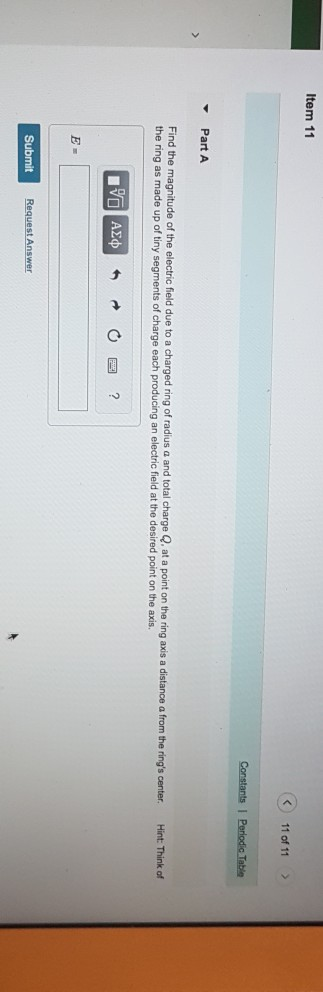# Question Solved1 AnswerItem 11 11 of 11> -Part A ring's center. Hint: Think of Find the magnitude of the electric field due to a charged ring of radius a and total charge Q, at a point on the ring axis a distance a from theTranscribed Image Text: Item 11 11 of 11> -Part A ring's center. Hint: Think of Find the magnitude of the electric field due to a charged ring of radius a and total charge Q, at a point on the ring axis a distance a from the
More
Transcribed Image Text: Item 11 11 of 11> -Part A ring's center. Hint: Think of Find the magnitude of the electric field due to a charged ring of radius a and total charge Q, at a point on the ring axis a distance a from the